# Relationship between WTI Crude Oil and Henry Hub Natural Gas¶

#### Author:¶

Rajan Subramanian
Commodity Quant Research Intern
Energy/Metals Group

#### Introduction¶

In [ ]:



This project examines the time series relationship between the Henry Hub Natural Gas price and the West Texas Intermediate (WTI) Crude Oil price. This relationship has been approached in the past using correlation and deterministic trends. Correlation is based on asset returns. Any analysis of correlation typically begins by detrending the price data. De-trending removes long term trend. Hence, it is impossible to base any decision on common trends in prices.

In the past, many studies have established that crude oil and gas prices are cointegrated. Yet recent studies have noted that the two price series have 'decoupled'. The prices have appeared to move independently of each other. Natural gas prices are more volatile (approximately twice as more) than Crude Oil Prices. According to recent literature, the co-integrating relationship doesn't seem stable over time. While rom 1989-2005, the two prices seemed to be trending upwards and co-integrated, after 2005, they two prices seems to have decoupled.

Economic theory suggests that the two commodities are related. They tend to be substitutes in production and consumption. Past changes in crude oil drove changes in natural gas prices, but the converse did not occur. This is because crude oil price is determined in the world market while natural gas market is regionally segmented. Hence, domestic natural gas market is much smaller than global crude oil market and hence less likely to influence the global price of oil.

The following are the economic factors linking Natural Gas and Crude Oil Market:

1) Increase in crude oil price motivates consumers to substitute nat gas for petroleum products in consumption which increases nat gas demand and hence prices.
2) Increase in crude oil price resulting from increase in crude oil demand may increase nat gas produced as a co-product of oil, which would decrease nat gas prices.
3) Increase in crude oil prices resulting from increase in crude oil demand may lead to increased costs of nat gas production and development, putting upward pressure on nat gas prices 4) Increase in crude oil prices resulting from an increase in crude oil demand may lead to more drilling and development of nat gas projects, which would tend to increase production and decrease nat gas prices.

#### Purpose¶

The purpose of this project is to better characterize the relationship between crude oil and natural gas. Specifically, we will do the following:

1) Examine the time series properties between natural gas and crude oil.
2) Estimate a VAR model to identify the fundamental tie (the cointegrating equation). This is the relationship that is generally established once two prices move away from each other 3) Determine the error correction mechanism. Whenever nat gas price has been pulled away from fundamental tie, the price will predictably, drift back to fundamental tie. This step estimates the rate at which the drift occurs 4)

#### Data Munging and Gathering¶

The data for daily Crude Oil and Natural Gas prices are obtained from the Bloomberg Terminal. Lets load the necessary libraries

In :
import numpy as np
import matplotlib.pyplot as plt
import pandas as pd
import seaborn as sns
import statsmodels.graphics.tsaplots as tsaplots
import statsmodels.api as sm
from pylab import rcParams
#some useful R import functions
import rpy2.robjects as robj
import pandas.rpy.common as com
from rpy2.robjects.packages import importr
import scipy.stats as sp
stats=importr('stats')
TSA = importr('TSA')
urca = importr('urca')
VARS = importr('vars')
%pylab inline
import stat2models as st2

Populating the interactive namespace from numpy and matplotlib

/usr/local/lib/python2.7/dist-packages/pandas/rpy/__init__.py:11: FutureWarning: The pandas.rpy module is deprecated and will be removed in a future version. We refer to external packages like rpy2.
See here for a guide on how to port your code to rpy2: http://pandas.pydata.org/pandas-docs/stable/r_interface.html
FutureWarning)


Now, we will import the necessary data which is stored in a excel file. Before we plot, lets munge the data to make the analysis more easier

In :
#read data from excel file


Plot of WTI Crude Oil and Natural Gas Raw Prices

In :
rcParams['figure.figsize'] = 12,4
axe = wti.plot(color='black')
we = natgas.plot(secondary_y=True,style='orange',ax=axe)
we.legend(loc='best')
axe.set_ylabel('Price (Dollars Per Barrel)')
axe.right_ax.set_ylabel('Price (Dollars per MMBtuu')
axe.set_title('Daily WTI/Nat Gas Prices')
axe.legend(loc='upper left')

Out:
<matplotlib.legend.Legend at 0x7fde1ecb1c50>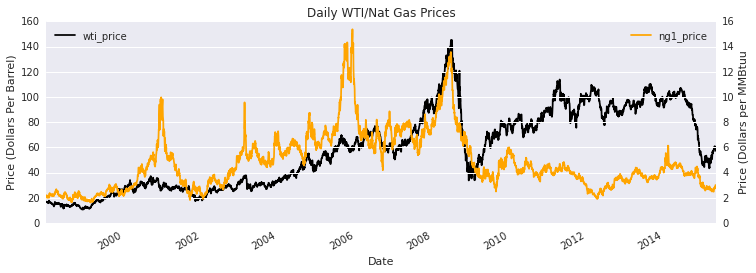#### Analysis¶

From the figure at the top, we can see that Nat Gas is more volatile than WTI. It seems that from just looking at the graphs, the correlation between WTI/Nat gas is high and positive. Since the data period includes daily prices, there might be too much noise in the daily data. Lets resample monthly. Monthly is used because they contain enough information to capture short-run movements over time. Hence, we will choose the first price of a particular month for the commodity. Also, log transformations is done for both the WTI and Nat Gas. Log Transformation is done because 1)Generally in Geometric Brownian Motion, it is:

$log(S_t / S_{t-1})$ ~ $N(\mu t,\sigma^2 t)$

that is, it is the log returns that are normally distributed. Also, 2) log returns are easier to work with since h period log return is sum of h-consequtive one period returns. This leads to square-root of time rule. 3) Log Transformations can remove scale effects and reduce possible effects of Heteroskedasticity.

Finally, as Crude Prices are generally higher than Nat Gas price by atleast a factor of 2, we will scale down the log price of WTI by subtracting the mean of log price of Nat Gas. This allows direct comparison of the two prices. That is:

$log(WTI_t) = log(WTI_t) - mean(log(Nat Gas_t))$

In [ ]:


In :
#resampling to monthly frequencies
#wti = wti.resample('W-MON',how='mean')
#natgas = natgas.resample('W-MON',how='mean')

#subtracting mean of log(Natgasprice) from log(wti)
Gt_wti = np.log(wti['wti_price'])-np.mean(np.log(natgas['ng1_price']))
xt_wti = Gt_wti.diff().dropna() #stores the log(returns of wti)
Gt_natgas = np.log(natgas['ng1_price'])
xt_natgas = Gt_natgas.diff().dropna() #stores the log(returns of nat gas)

In :
#plotting the data
rcParams['figure.figsize'] = 12,4
plt.plot(Gt_wti.index,Gt_wti.values,color='black',label='WTI Crude Oil Log(Price)')
plt.plot(Gt_natgas.index,Gt_natgas.values,color='orange',label='HenryHub Natural Gas Price (log Price)')
plt.legend(loc='best')
plt.ylabel('log price (Dollars)')
plt.xlabel('Date')
plt.title('Daily Nat Gas Log Prices & Mean-Adjusted log(WTI) 01/29 /1998-5/20/2015')

Out:
<matplotlib.text.Text at 0x7fde1f1e6e10>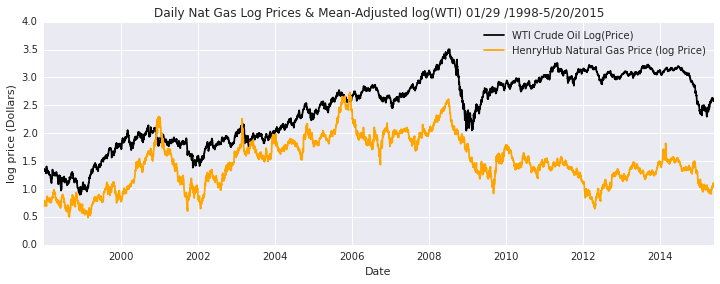###### Analysis¶

From visual inspection, we can see that nat gas prices are more volatile than crude oil price.
%R cat('ng1 order select', ar(y_ng_ret,demean=FALSE)$order)  wti order select 6 ng1 order select 1 We can see that the automatic selection picks an AR(6) for the log (returns) of wti and AR(1) for logNatGas. This confirms with the initial observation of the pacf graph that the order of the model is of AR(1) & AR(9) respectively. Lets check the residuals of the model: In : rcParams['figure.figsize'] = 11,8 fig,axes = plt.subplots(2,1) model_wti1 = sm.tsa.ARMA(xt_wti.values,order=(9,0)).fit(trend='nc') #based on PACF criteria. AIC leads to corr at lag 13 model_natgas = sm.tsa.ARMA(xt_natgas.values,order=(10,0)).fit(trend='nc') #based on PACF only tsaplots.plot_acf(model_wti1.resid,lags=24,ax=axes); tsaplots.plot_acf(model_natgas.resid,lags=24,ax=axes); axes.set_title('ACF log(WTI returns) residuals') axes.set_title('ACF log(NatGas Returns) residuals ')  Out: <matplotlib.text.Text at 0x7fde1557b3d0>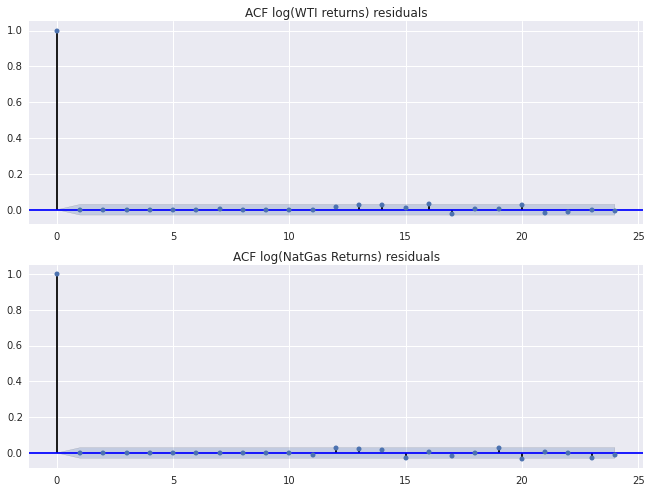##### Analysis¶ We can see that the two models for wti and natgas fits pretty well. The ACFs of the residuals don't show any significant lags at 5% significance. Although in the wti, there seems to be a minor significance around lag 13. But this is to be expected since in a sample size of 100, there is a 5% chance that our residuals will be correlated. In [ ]:   In : #ljung box test on the residuals print "ljung box test on wti residuals \n" st2.boxTest(model_wti1.resid) print '\n' print "ljung box test on nat gas residuals \n" st2.boxTest(model_natgas.resid)  ljung box test on wti residuals Xsquared 0.0440559665223 pvalue 0.999999999958 Xsquared 17.3472495506 pvalue 0.630323779628 ljung box test on nat gas residuals Xsquared 0.0201591143789 pvalue 0.999999999999 Xsquared 19.7019525422 pvalue 0.476707367819  #### ADF Test & Determining the trend and intercept for WTI and NatGas¶ We perform two forms of ADF test due to viual observation of the data. Each form differs based on deterministic component of the trend:$\delta x_t = \beta_0 + \phi_1 x_{t-1} + \sum\limits_{i=0}^{p}(\gamma_i \delta x_{t-i}) + \epsilon_t\delta x_t = \beta_0 + \beta_1 t + \phi_1 x_{t-1} + \sum\limits_{i=0}^{p}(\gamma_i \delta x_{t-i}) + \epsilon_t$Following Elder/Kennedy's methodology to determine if there is a trend or intercept in the data: #### Growth of the series is not known:¶ Assume our regression is of the form:$\delta x_t = \beta_0 + \beta_1 t + \phi_1 x_{t-1} + \sum\limits_{i=0}^{p}(\gamma_i \delta x_{t-i}) + a_t$Ignore the fact that$\beta_1$is 0. We will conduct the ADF to test for stationarity. If the data is not stationary,$\beta_1$= 0 would follow. Since there is unit root, we test presence of intercept by regressing$\delta x_t$against the remaining variables on the rhs of above equation ##### ADF test¶ In : st2.print_adfuller(Gt_wti.values,title='Log(WTI)-based on PACF',maxlag=9,regression='ct') # Based on PACF observation print('\n') st2.print_adfuller(Gt_wti.values,title='Log(WTI)-based on t-stat',regression='ct',autolag='t-stat') # Based on t-stat print('\n') st2.print_adfuller(Gt_wti.values,title='Log(WTI)-based on AIC',regression='ct',autolag='AIC') # Based on t-stat print('\n') st2.print_adfuller(xt_wti.values,title='Log(WTI) Returns',maxlag=9,regression='nc') #checking if returns are stationary print('\n') st2.print_adfuller(Gt_natgas.values,title='Log(NatGas) based on PACF',maxlag=10,regression='ct') #Based on PACF observation print('\n') st2.print_adfuller(Gt_natgas.values,title='Log(NatGas) based on t-stat',regression='ct',autolag='t-stat') print('\n') st2.print_adfuller(Gt_natgas.values,title='Log(NatGas) based on AIC',regression='ct',autolag='AIC') print('\n') st2.print_adfuller(xt_natgas.values,title='Log(WTI) Returns',maxlag=10,regression='nc')  Augmented Dickey Fuller Test commodity : Log(WTI)-based on PACF Dickey-Fuller = -1.63181410507 Lag order = 9 p value = 0.779727778289 alternative hypothesis: stationary Augmented Dickey Fuller Test commodity : Log(WTI)-based on t-stat Dickey-Fuller = -2.05244956839 Lag order = 27 p value = 0.572598782289 alternative hypothesis: stationary Augmented Dickey Fuller Test commodity : Log(WTI)-based on AIC Dickey-Fuller = -1.75937402162 Lag order = 6 p value = 0.724010223777 alternative hypothesis: stationary Augmented Dickey Fuller Test commodity : Log(WTI) Returns Dickey-Fuller = -22.2735916248 Lag order = 9 p value = 0.0 alternative hypothesis: stationary Augmented Dickey Fuller Test commodity : Log(NatGas) based on PACF Dickey-Fuller = -2.46585523458 Lag order = 10 p value = 0.345238275513 alternative hypothesis: stationary Augmented Dickey Fuller Test commodity : Log(NatGas) based on t-stat Dickey-Fuller = -2.30604490265 Lag order = 27 p value = 0.430609222673 alternative hypothesis: stationary Augmented Dickey Fuller Test commodity : Log(NatGas) based on AIC Dickey-Fuller = -2.46008646895 Lag order = 1 p value = 0.348196213139 alternative hypothesis: stationary Augmented Dickey Fuller Test commodity : Log(WTI) Returns Dickey-Fuller = -20.1005645203 Lag order = 10 p value = 0.0 alternative hypothesis: stationary  In [ ]:   ##### Analysis¶ Hence we see that presence of unit root is not rejected. i.e, we accept that the series is not stationary. Our testing of stationarity is done. Hence$\beta_1$= 0. Next step, we test presence of intercept by regressing change in series against intercept:$\delta x_t = \beta_0 + \sum\limits_{i=0}^{p}(\gamma_i \delta x_{t-i}) + a_t$Notice...this is just a traditional AR model on the differences...Hence, we fit an AR(6) model for wti and AR(1) for nat_gas to determine the t statistic on the coefficient. We can run this regression because the log(returns) are stationary In : m1 = st2.ar_ols(xt_wti.values,lags=9) m2 = st2.ar_ols(xt_natgas.values,lags=10) print 'wti_const = ', m1.params print 'wti_p value = ', m1.pvalues print '\n' print 'natgas_const = ', m2.params print 'natgas_p value = ', m2.pvalues  wti_const = 0.000332944049915 wti_p value = 0.363800928384 natgas_const = 7.92473967396e-05 natgas_p value = 0.880670352897  Hence we accept that the drift is 0 and both of them have no trend. Hence, both series are I(1) with no drift or trending terms In : #finally, using phillips perrons test to confirm that the series is stationary: from arch.unitroot import PhillipsPerron print "wti prices" print(PhillipsPerron(Gt_wti,trend='nc',lags=9)) print '\n' print 'nat gas prices' print(PhillipsPerron(Gt_natgas,trend='nc',lags=10))  wti prices Phillips-Perron Test (Z-tau) ===================================== Test Statistic 0.360 P-value 0.791 Lags 9 ------------------------------------- Trend: No Trend Critical Values: -2.57 (1%), -1.94 (5%), -1.62 (10%) Null Hypothesis: The process contains a unit root. Alternative Hypothesis: The process is weakly stationary. nat gas prices Phillips-Perron Test (Z-tau) ===================================== Test Statistic -0.619 P-value 0.447 Lags 10 ------------------------------------- Trend: No Trend Critical Values: -2.57 (1%), -1.94 (5%), -1.62 (10%) Null Hypothesis: The process contains a unit root. Alternative Hypothesis: The process is weakly stationary.  The Phillips Perron test also confirm that the original series is I(1) for both the series ## Part II: Co-Integration Analysis¶ two time Series$X_t$and$Y_t$are co-integrated if each is I(1) and there exists a$\lambda$such that:$Z = X_t -\lambda Y_t $is stationary. The process$Z_t$is called disequilibrium because it captures deviations from long term equilibrium. Generally, price data is detrended even before any analysis even begins. Hence, long term trend is generally removed. Hence, it impossible to base any decision on common stochastic trends. In co-integration, we have to first test if theres a common stochastic trends in variables. If there is a common stochsic trend in set of prices, they must have long term equilibrium relationship. Second goal of cointegration analysis is to capture this equilibrium in a dynamic correlation analysis. Hence, we have a two set procedure: a) long term equilibrium relationship between prices is established. Statistical tests for cointegration is applied, and if cointegration is present, we identifuy stationary linear combination of prices which best describes long term equilibrum relationship between them. b) long term equilibrium is used in error correction model (ECM) of returns. ECMs explain how short term deviations from equilibrium are corrected. ##### a) Establishing Long Term Relationship (Engle-Granger Methodology)¶ We can see from the very first picture on the top, that the two series seems co-integrated. Following is a scatter plot of the two integrated Series and a regression model regressing Log(nat gas price) against log(crude oil price) :$log(natgas_t) = \beta_0 + \beta_1 log(wti_t) + \epsilon_t$The above regression model determines whether the two series are co-integrated because once we run the regression and fit the model, then we subtract the log of WTI from log of nat gas, that is:$log(natgas_t) - \beta_1 log(wti_t) - \beta_0 = \epsilon_t$Hence,$ \beta_1 $determines the parameter needed to form a stationarity between the two series. Hence, from the rhs of the equation, an observation of the residuals would indicate whether the series is stationary or not. Engle Granger propose applying unit root testing on the residuals from the static regression above. Unit root test regression on the residuals is without the deterministic terms (constant + trend). According to Phillips and Ouliaris, ADF and Phillips Perrons Unit tests as applied to the residuals do not follow the usual Dickey Fuller Distribution. But, due to spurious regression, distribution of ADF and PP test has asymptotic distirbutions that are a function of Weirner Process that depend on the deterministic terms and number of variables used as the dependent variable. Hence, we use the Phillips Ouliaris test on the residuals to determine if the two series are co-integrating or not. Also, due to Hansen (1992), PO distributions of the ADF and PP test applied to residuals depend on the trend behavior as follows: 1) if two series are both I(1) without drift, then use regression with just a constant with dimension parameter n-1 2) if independent variable is I(1) with drift while the dependent one may or maynot be I(1) with drift, use regression with constant plus trend in PO test with dimension parameter n-2. If n-2 = 0, then use DF distribution adjusted for constant and trend 3) Dependent is I(1) with drift and indepdent is I(1) without drift. Use constnat plus trend in regression. Use PO test with dimension n-1 Since we already know that both our series are I(1) without the drift, we will use the fist criteria and just specify the constant: In : prices = ['log(natgas)','log(wti)'] #empirically, wti causes nat gas Gt_data = pd.DataFrame(np.column_stack([Gt_natgas.values,Gt_wti.values]),index=Gt_natgas.index,columns=prices) xt_data = Gt_data.diff().dropna() returns = ['log(natgas)return','log(wti)return'] xt_data.columns = returns r_df = com.convert_to_r_dataframe(Gt_data) %Rpush r_df %R y = ts(r_df) %R print(summary(ca.po(y,lag='long',type='Pz',demean='constant')))  ######################################## # Phillips and Ouliaris Unit Root Test # ######################################## Test of type Pz detrending of series with constant only Response log.natgas. : Call: lm(formula = log.natgas. ~ zr) Residuals: Min 1Q Median 3Q Max -0.19643 -0.01977 -0.00053 0.01854 0.32584 Coefficients: Estimate Std. Error t value Pr(>|t|) (Intercept) 0.0040358 0.0022919 1.761 0.0783 . zrlog.natgas. 0.9965988 0.0013057 763.244 <2e-16 *** zrlog.wti. 0.0004305 0.0009415 0.457 0.6475 --- Signif. codes: 0 ‘***’ 0.001 ‘**’ 0.01 ‘*’ 0.05 ‘.’ 0.1 ‘ ’ 1 Residual standard error: 0.03485 on 4356 degrees of freedom Multiple R-squared: 0.9941, Adjusted R-squared: 0.9941 F-statistic: 3.658e+05 on 2 and 4356 DF, p-value: < 2.2e-16 Response log.wti. : Call: lm(formula = log.wti. ~ zr) Residuals: Min 1Q Median 3Q Max -0.166412 -0.012778 0.000534 0.013249 0.163391 Coefficients: Estimate Std. Error t value Pr(>|t|) (Intercept) 2.871e-03 1.589e-03 1.806 0.0709 . zrlog.natgas. 2.792e-05 9.055e-04 0.031 0.9754 zrlog.wti. 9.989e-01 6.530e-04 1529.824 <2e-16 *** --- Signif. codes: 0 ‘***’ 0.001 ‘**’ 0.01 ‘*’ 0.05 ‘.’ 0.1 ‘ ’ 1 Residual standard error: 0.02417 on 4356 degrees of freedom Multiple R-squared: 0.9985, Adjusted R-squared: 0.9985 F-statistic: 1.469e+06 on 2 and 4356 DF, p-value: < 2.2e-16 Value of test-statistic is: 24.5533 Critical values of Pz are: 10pct 5pct 1pct critical values 47.5877 55.2202 71.9273  The two series is not co-integrated according to the Phillips Ouliaris test. The PO test gives a test statistic of 24.5729 which is less than the 10% critical value of 44.8877. Hence, we accept that the two series are not co-integrated, at least in this data period. Next we plot the residuals from our static regression and also show the acf and pacf plots: In : rcParams['figure.figsize'] = 14,3 fig,axes = plt.subplots(1,3) n = len(Gt_natgas) t = np.arange(0,n) X = np.column_stack((np.ones(n),Gt_wti.values)) res = sm.OLS(Gt_natgas.values,X).fit() # includes a constant: y(t) = a + bx(t) + e_t axes.plot(res.resid,color='firebrick') axes.set_title('residual plot') tsaplots.plot_acf(res.resid,lags=24,ax=axes); axes.set_title('ACF residuals') tsaplots.plot_pacf(res.resid,lags=24,ax=axes); axes.set_title('PACF residuals')  Out: <matplotlib.text.Text at 0x7fde15142310>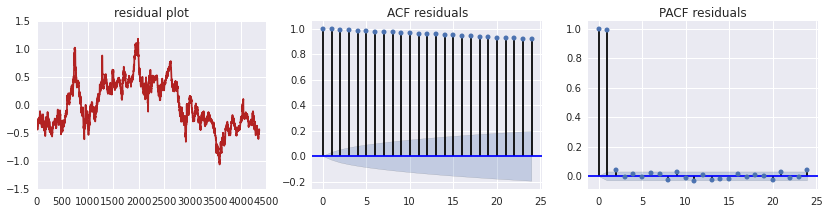#### Analysis¶ The above graph plots the residual plot from the co-integrating regression (with a constant) and includes the ACF and PACF graph respectively. We can see a slowly declining ACF for the residual and a sudden decline in PACF. From the plots, it doesn't look like the residual series is stationary. In : from statsmodels.stats.stattools import durbin_watson print "Rsquared: ", res.rsquared print "DW statistic: ", durbin_watson(res.resid)  Rsquared: 0.203251952962 DW statistic: 0.00696216809669  ### Analysis¶ We notice a small DW statistic and low Rsquared. ACF plot of residuals shows a slowly declining correlation. Phillips Ouliaris test shows that the data is not cointegrated. Hence, the parameters obtained for the static regression model is spurious or non sense. This is in essence, the Engle-Granger Methodology. The methodology says that: 1)Test inidividual variables$X_{t}$and$Y_{t}$for unit roots 2)Run the static cointegrating regression:$Y_{t} = \beta_0 + \beta_1 X_{t} + \epsilon_t $3) Test for no-cointegration by testing for unit root in residuals,$\epsilon_t $. Unit root regression for residuals is without the deterministic terms (constant or constant and trend) 4) If co-integration is not rejected, check for a dynamic ECM model. We apply the OLS regression of one integrated variable on another integrated variable and apply Engle Granger test on the residuals. However, Phillips and Ouliaris show that ADF root tests applied to estimated cointegrating residual do not have the usual Dickey Fuller distribution under null of no cointegration. These distributions depend on the Phillips-Ouliaris (PO) distributions. We can also confirm using the Phillips-Ouliaris co-integration (which is an implementation of Engle-Granger Methodology and an improvement over EG methodology for co-integration) test. Finally, before we proceed to these testing...we will cover Johansen Procedure which is another form of co-integration testing... #### Johansen Procedure¶ The basic steps are the following: 1) Specify and Estimate a VAR(p) model for$Y_t$2) Construct likelihood ratio tests for rank of coefficient matrix to determine number of co-integrating vector 3) Impose normalization and identify restrictions on co-integrating vectors 4) Given cointegrating vector, estimate the cointegrated VECM using Maximum likelihood Again, there are special cases that depends on whether the data has a restricted constant or trending. Refer to Eric Zivot's chapter 12 -Cointegration In : %R print(summary(ca.jo(y,type='eigen',ecdet='const'))) %R print(summary(ca.jo(y,type='trace',ecdet='const')))  ###################### # Johansen-Procedure # ###################### Test type: maximal eigenvalue statistic (lambda max) , without linear trend and constant in cointegration Eigenvalues (lambda):  1.568201e-03 8.862071e-04 2.168404e-19 Values of teststatistic and critical values of test: test 10pct 5pct 1pct r <= 1 | 3.86 7.52 9.24 12.97 r = 0 | 6.84 13.75 15.67 20.20 Eigenvectors, normalised to first column: (These are the cointegration relations) log.natgas..l2 log.wti..l2 constant log.natgas..l2 1.0000000 1.000000 1.000000 log.wti..l2 -0.1592085 -2.656973 -4.149159 constant -1.1045779 5.654689 3.126602 Weights W: (This is the loading matrix) log.natgas..l2 log.wti..l2 constant log.natgas..d -0.003289303 0.0000507866 1.792455e-19 log.wti..d -0.000399533 0.0004266265 -7.171711e-20  ###################### # Johansen-Procedure # ###################### Test type: trace statistic , without linear trend and constant in cointegration Eigenvalues (lambda):  1.568201e-03 8.862071e-04 2.168404e-19 Values of teststatistic and critical values of test: test 10pct 5pct 1pct r <= 1 | 3.86 7.52 9.24 12.97 r = 0 | 10.70 17.85 19.96 24.60 Eigenvectors, normalised to first column: (These are the cointegration relations) log.natgas..l2 log.wti..l2 constant log.natgas..l2 1.0000000 1.000000 1.000000 log.wti..l2 -0.1592085 -2.656973 -4.149159 constant -1.1045779 5.654689 3.126602 Weights W: (This is the loading matrix) log.natgas..l2 log.wti..l2 constant log.natgas..d -0.003289303 0.0000507866 1.792455e-19 log.wti..d -0.000399533 0.0004266265 -7.171711e-20  #### Analysis¶ There is no co-integration between 1998-2015. You cannot apply ADF test on the residuals. Testing stationarity of residuals follows the phillipps-Ouliaris distribution. According to the po test above, it has the benefit that it doesn't matter what the dependent variable in the regression equation is. We can clearly see that, including a constant in our regression stills leads to no co-integration. We can conclude, that for period 1998-2015, Crude WTI/NatGas is not co-integrated. We can see that, it seems structural breaks in the data might affect the outcome of the test. It looks like from 2010, there was a structural break in the data where the two prices have diverged. Perhaps more time needs to pass to see whether the relationship eventually co-integrates. Also, some of these tests that perform ADF tests on the residuals are too sensitive to the tests at hand. For example, recall that an AR(1) is stationary if and only if$ \beta_1 $is$<= |1|$:$x_t$=$\beta_0 + \beta_1 x_{t-1} + \epsilon_t$. Subtracting we find..$\delta x_t $=$\beta_0 + x_{t-1}\pi + \epsilon_t $where$\pi$= ($\beta_1 - 1$) Hence, testing for unit root is analogous to testing for$\pi$= 0. If the number is very close to 0, i.e 0.000056 etc..there is nothing to distinguish it from 0 and not 0. Bottom line is if roots are close to unity, we might have tests that fail and others that pass #### TimeSpan¶ According to literature, Crude Oil WTI and Natgas were co-integrated in the past. Looking at the figure, we can see that a possible relationship might have existed from 1998-2009. After 2009, it looks like the spread between the two commodities have increased. Lets run our tests on these dates: 1998-2006 1998-01/2009 2009-current In : def filter_data(wti,natgas,start,end): #return the wti and natgas log prices corresponding to the start and end dates x = np.log(wti.ix[start:end,'wti_price']) - np.mean(np.log(natgas.ix[start:end,'ng1_price'])) y = np.log(natgas.ix[start:end,'ng1_price']) return x,y  # Eye Test¶ In : plt.plot(Gt_data.values) plt.plot((3045,3045),(0,4),'r-') plt.plot((2620,2620),(0,4),color='orange') plt.plot((2760,2760),(0,4),'b-') print(Gt_data.index) print(Gt_data.index) print(Gt_data.index)  2010-03-04 00:00:00 2008-06-25 00:00:00 2009-01-14 00:00:00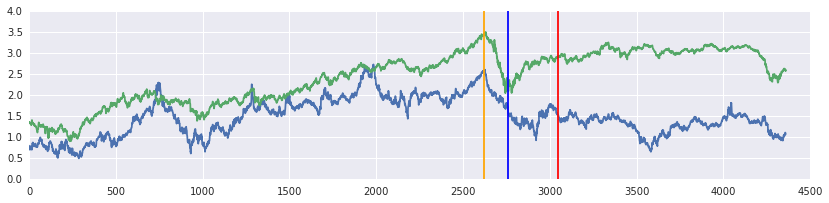Red line marks the end of co-integrating relationship (done through trial and error) Blue line indicates the beginning of decoupling effect Orange line marks the beginning of financial crisis In : crude,ng1 = filter_data(wti,natgas,start='1998',end='03-04-2010') #filter the time period. From 06-29-2009: no co-integration fig,ax = plt.subplots(1,2) rcParams['figure.figsize'] = 12,5 tsaplots.plot_pacf(crude.diff().dropna(),lags=24,ax=ax); #plot the pacf for the two commodites tsaplots.plot_pacf(ng1.diff().dropna(),lags=24,ax=ax);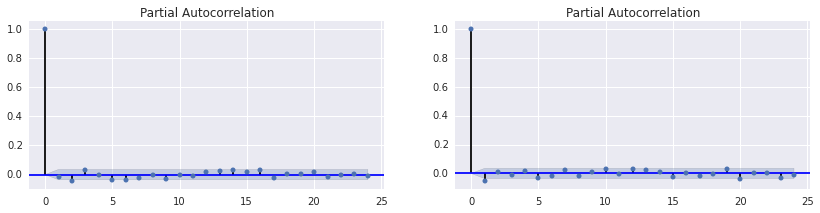In [ ]:   In : from arch.unitroot import ADF #based on Enders/kennedy method to determine if data has a drift + trend. print(ADF(crude.values,trend='ct',lags=9)) #based on pacf print '\n' print(ADF(ng1.values,trend='ct',lags=5))   Augmented Dickey-Fuller Results ===================================== Test Statistic -2.402 P-value 0.378 Lags 9 ------------------------------------- Trend: Constant and Linear Time Trend Critical Values: -3.96 (1%), -3.41 (5%), -3.13 (10%) Null Hypothesis: The process contains a unit root. Alternative Hypothesis: The process is weakly stationary. Augmented Dickey-Fuller Results ===================================== Test Statistic -2.379 P-value 0.391 Lags 5 ------------------------------------- Trend: Constant and Linear Time Trend Critical Values: -3.96 (1%), -3.41 (5%), -3.13 (10%) Null Hypothesis: The process contains a unit root. Alternative Hypothesis: The process is weakly stationary.  In : #we accept the data is integrated. Hence, beta_1 = 0. Now we regress difference with just constant: m1 = st2.ar_ols(crude.diff().dropna(),9) m2 = st2.ar_ols(ng1.diff().dropna(),5) print 'wti_const = ', m1.params print 'wti_p value = ', m1.pvalues print '\n' print 'natgas_const = ', m2.params print 'natgas_p value = ', m2.pvalues  wti_const = 0.000612007260768 wti_p value = 0.200041037015 natgas_const = 0.000293656599387 natgas_p value = 0.666964645193  In : #accept that there is no intercept. Next we do the ljung box test to check validity of the model: st2.boxTest(m1.resid)  Xsquared 0.0742197904134 pvalue 0.999999999431 Xsquared 13.6090348936 pvalue 0.849752840782  In : st2.boxTest(m2.resid)  Xsquared 6.39847540417 pvalue 0.780748257887 Xsquared 21.839151329 pvalue 0.349302150232  Hence, no trend and no constant in our model. We accept that the data is integrated during this time period as well. Ljung Box test on the residuals don't show any correlation for both the shorter and longer lags. We accept there is no correlation in the residuals. We confirm using Phillips Perrons Unit test: In : print(PhillipsPerron(crude.values,lags=9,trend='nc')) print '\n' print(PhillipsPerron(ng1.values,lags=5,trend='nc'))   Phillips-Perron Test (Z-tau) ===================================== Test Statistic 0.783 P-value 0.882 Lags 9 ------------------------------------- Trend: No Trend Critical Values: -2.57 (1%), -1.94 (5%), -1.62 (10%) Null Hypothesis: The process contains a unit root. Alternative Hypothesis: The process is weakly stationary. Phillips-Perron Test (Z-tau) ===================================== Test Statistic -0.346 P-value 0.558 Lags 5 ------------------------------------- Trend: No Trend Critical Values: -2.57 (1%), -1.94 (5%), -1.62 (10%) Null Hypothesis: The process contains a unit root. Alternative Hypothesis: The process is weakly stationary.  In : #Next we perform Phillips Ouliaris test and Johansen's test: df_pre = pd.DataFrame(np.column_stack([ng1.values,crude.values]),index=ng1.index,columns=prices) r_df = com.convert_to_r_dataframe(df_pre) %Rpush r_df %R y.pre = ts(r_df) %R print(summary(ca.po(y.pre,lag='long',type='Pz',demean='constant'))) #phillps ouliaris test  ######################################## # Phillips and Ouliaris Unit Root Test # ######################################## Test of type Pz detrending of series with constant only Response log.natgas. : Call: lm(formula = log.natgas. ~ zr) Residuals: Min 1Q Median 3Q Max -0.19426 -0.02135 -0.00027 0.01956 0.32696 Coefficients: Estimate Std. Error t value Pr(>|t|) (Intercept) 0.002412 0.002534 0.952 0.3413 zrlog.natgas. 0.992650 0.002352 422.062 <2e-16 *** zrlog.wti. 0.004392 0.002015 2.180 0.0294 * --- Signif. codes: 0 ‘***’ 0.001 ‘**’ 0.01 ‘*’ 0.05 ‘.’ 0.1 ‘ ’ 1 Residual standard error: 0.0376 on 3042 degrees of freedom Multiple R-squared: 0.9945, Adjusted R-squared: 0.9945 F-statistic: 2.73e+05 on 2 and 3042 DF, p-value: < 2.2e-16 Response log.wti. : Call: lm(formula = log.wti. ~ zr) Residuals: Min 1Q Median 3Q Max -0.166191 -0.014278 0.000604 0.015016 0.163356 Coefficients: Estimate Std. Error t value Pr(>|t|) (Intercept) 0.0027453 0.0017716 1.55 0.121 zrlog.natgas. 0.0003775 0.0016440 0.23 0.818 zrlog.wti. 0.9986609 0.0014086 708.99 <2e-16 *** --- Signif. codes: 0 ‘***’ 0.001 ‘**’ 0.01 ‘*’ 0.05 ‘.’ 0.1 ‘ ’ 1 Residual standard error: 0.02629 on 3042 degrees of freedom Multiple R-squared: 0.998, Adjusted R-squared: 0.998 F-statistic: 7.642e+05 on 2 and 3042 DF, p-value: < 2.2e-16 Value of test-statistic is: 49.7624 Critical values of Pz are: 10pct 5pct 1pct critical values 47.5877 55.2202 71.9273  Phillips Ouliaris test gives a test statistic of 49.1058. The 10% and 5 % critical values are 47.5877 and 55.2202 respectively. Hence, the PO test rejects the null of no cointegration. Hence, we can be 90% confident, that the two series were co-integrated in this time period. However, the test fails at the 5% and 1% significance. In : #Johansen test: %R print(summary(ca.jo(y.pre,type='eigen',ecdet='const'))) %R print(summary(ca.jo(y.pre,type='trace',ecdet='const')))  ###################### # Johansen-Procedure # ###################### Test type: maximal eigenvalue statistic (lambda max) , without linear trend and constant in cointegration Eigenvalues (lambda):  3.571009e-03 9.644994e-04 1.734723e-18 Values of teststatistic and critical values of test: test 10pct 5pct 1pct r <= 1 | 2.94 7.52 9.24 12.97 r = 0 | 10.89 13.75 15.67 20.20 Eigenvectors, normalised to first column: (These are the cointegration relations) log.natgas..l2 log.wti..l2 constant log.natgas..l2 1.0000000 1.000000 1.00000 log.wti..l2 -0.6692787 -5.942049 -18.28086 constant -0.1539628 13.531631 24.46925 Weights W: (This is the loading matrix) log.natgas..l2 log.wti..l2 constant log.natgas..d -0.0073773112 8.752692e-05 -3.197957e-19 log.wti..d 0.0001103594 2.026133e-04 -1.094570e-20  ###################### # Johansen-Procedure # ###################### Test type: trace statistic , without linear trend and constant in cointegration Eigenvalues (lambda):  3.571009e-03 9.644994e-04 1.734723e-18 Values of teststatistic and critical values of test: test 10pct 5pct 1pct r <= 1 | 2.94 7.52 9.24 12.97 r = 0 | 13.83 17.85 19.96 24.60 Eigenvectors, normalised to first column: (These are the cointegration relations) log.natgas..l2 log.wti..l2 constant log.natgas..l2 1.0000000 1.000000 1.00000 log.wti..l2 -0.6692787 -5.942049 -18.28086 constant -0.1539628 13.531631 24.46925 Weights W: (This is the loading matrix) log.natgas..l2 log.wti..l2 constant log.natgas..d -0.0073773112 8.752692e-05 -3.197957e-19 log.wti..d 0.0001103594 2.026133e-04 -1.094570e-20  #### Analysis¶ Johansen's test gives a value of 9.41 and hence fails to reject no cointegration. Finally, Johansen's trace fails to accept that the data is cointegrated. Quite Possible that during this time, there is a possibility of cointegration or if it existed, it was weak. ## 04/2010-05/20/2015¶ In : crude,ng1 = filter_data(wti,natgas,start='04-2010',end='05-20-2015') #filter the time period fig,ax = plt.subplots(1,2) rcParams['figure.figsize'] = 12,4 tsaplots.plot_pacf(crude.diff().dropna(),lags=24,ax=ax); #plot the pacf for the two commodites tsaplots.plot_pacf(ng1.diff().dropna(),lags=24,ax=ax);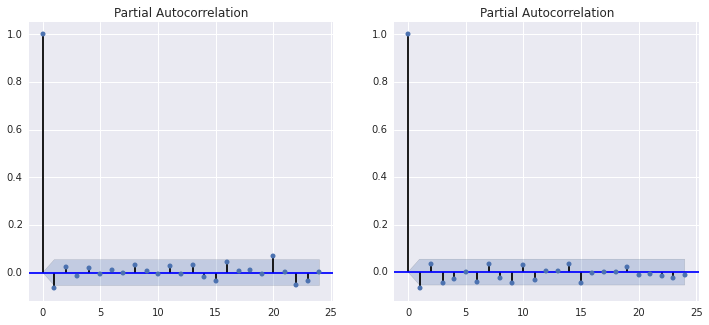In : print(ADF(crude.values,trend='ct',lags=1)) #based on pacf print '\n' print(ADF(ng1.values,trend='ct',lags=1))   Augmented Dickey-Fuller Results ===================================== Test Statistic -1.214 P-value 0.908 Lags 1 ------------------------------------- Trend: Constant and Linear Time Trend Critical Values: -3.97 (1%), -3.41 (5%), -3.13 (10%) Null Hypothesis: The process contains a unit root. Alternative Hypothesis: The process is weakly stationary. Augmented Dickey-Fuller Results ===================================== Test Statistic -2.223 P-value 0.477 Lags 1 ------------------------------------- Trend: Constant and Linear Time Trend Critical Values: -3.97 (1%), -3.41 (5%), -3.13 (10%) Null Hypothesis: The process contains a unit root. Alternative Hypothesis: The process is weakly stationary.  In : #Hence we accept that the two time series are integrated. Next we specify the model with a constant: m1 = st2.ar_ols(crude.diff().dropna(),1) m2 = st2.ar_ols(ng1.diff().dropna(),1) print 'wti_const = ', m1.params print 'wti_p value = ', m1.pvalues print '\n' print 'natgas_const = ', m2.params print 'natgas_p value = ', m2.pvalues  wti_const = -0.000320841124112 wti_p value = 0.530964229016 natgas_const = -0.000315141428769 natgas_p value = 0.67879035658  In : #again, no constant, and no trend in our data. Next we perform PhillipsPerron unit test: print(PhillipsPerron(crude.values,trend='nc')) print '\n' print(PhillipsPerron(ng1.values,trend='nc'))   Phillips-Perron Test (Z-tau) ===================================== Test Statistic -0.613 P-value 0.449 Lags 23 ------------------------------------- Trend: No Trend Critical Values: -2.57 (1%), -1.94 (5%), -1.62 (10%) Null Hypothesis: The process contains a unit root. Alternative Hypothesis: The process is weakly stationary. Phillips-Perron Test (Z-tau) ===================================== Test Statistic -0.703 P-value 0.411 Lags 23 ------------------------------------- Trend: No Trend Critical Values: -2.57 (1%), -1.94 (5%), -1.62 (10%) Null Hypothesis: The process contains a unit root. Alternative Hypothesis: The process is weakly stationary.  In : st2.boxTest(m1.resid)  Xsquared 3.50661264242 pvalue 0.966873374825 Xsquared 17.0178368508 pvalue 0.651815132571  In : st2.boxTest(m2.resid)  Xsquared 12.8612503594 pvalue 0.231533281821 Xsquared 20.6759900301 pvalue 0.416419172152  In : #perform the phillips ouliaris test and johanset test df_post = pd.DataFrame(np.column_stack([ng1.values,crude.values]),index=ng1.index,columns=prices) r_df = com.convert_to_r_dataframe(df_post) %Rpush r_df %R y.post = ts(r_df) %R print(summary(ca.po(y.post,lag='long',type='Pz',demean='constant'))) #phillips perron unit test  ######################################## # Phillips and Ouliaris Unit Root Test # ######################################## Test of type Pz detrending of series with constant only Response log.natgas. : Call: lm(formula = log.natgas. ~ zr) Residuals: Min 1Q Median 3Q Max -0.11473 -0.01701 -0.00024 0.01623 0.16652 Coefficients: Estimate Std. Error t value Pr(>|t|) (Intercept) -0.0006415 0.0127135 -0.050 0.960 zrlog.natgas. 0.9906251 0.0037985 260.796 <2e-16 *** zrlog.wti. 0.0039183 0.0040469 0.968 0.333 --- Signif. codes: 0 ‘***’ 0.001 ‘**’ 0.01 ‘*’ 0.05 ‘.’ 0.1 ‘ ’ 1 Residual standard error: 0.02738 on 1291 degrees of freedom Multiple R-squared: 0.9824, Adjusted R-squared: 0.9824 F-statistic: 3.601e+04 on 2 and 1291 DF, p-value: < 2.2e-16 Response log.wti. : Call: lm(formula = log.wti. ~ zr) Residuals: Min 1Q Median 3Q Max -0.108039 -0.009506 0.000511 0.009782 0.089230 Coefficients: Estimate Std. Error t value Pr(>|t|) (Intercept) 0.0090995 0.0085643 1.062 0.288 zrlog.natgas. -0.0005765 0.0025588 -0.225 0.822 zrlog.wti. 0.9972892 0.0027261 365.830 <2e-16 *** --- Signif. codes: 0 ‘***’ 0.001 ‘**’ 0.01 ‘*’ 0.05 ‘.’ 0.1 ‘ ’ 1 Residual standard error: 0.01845 on 1291 degrees of freedom Multiple R-squared: 0.991, Adjusted R-squared: 0.9909 F-statistic: 7.071e+04 on 2 and 1291 DF, p-value: < 2.2e-16 Value of test-statistic is: 19.89 Critical values of Pz are: 10pct 5pct 1pct critical values 47.5877 55.2202 71.9273  #### Observation¶ PO test has failed to reject the null of no cointegration. i.e the data is not co-integrated during this time period. Next we perform the Johansen test: In : #Johansen test: %R print(summary(ca.jo(y.post,type='eigen',ecdet='const'))) %R print(summary(ca.jo(y.post,type='trace',ecdet='const')))  ###################### # Johansen-Procedure # ###################### Test type: maximal eigenvalue statistic (lambda max) , without linear trend and constant in cointegration Eigenvalues (lambda):  4.559679e-03 9.744094e-04 2.806730e-19 Values of teststatistic and critical values of test: test 10pct 5pct 1pct r <= 1 | 1.26 7.52 9.24 12.97 r = 0 | 5.91 13.75 15.67 20.20 Eigenvectors, normalised to first column: (These are the cointegration relations) log.natgas..l2 log.wti..l2 constant log.natgas..l2 1.0000000 1.000000 1.000000 log.wti..l2 -0.4812282 8.184129 1.935679 constant 0.2732822 -26.301218 -8.135502 Weights W: (This is the loading matrix) log.natgas..l2 log.wti..l2 constant log.natgas..d -0.0088752672 -3.343469e-05 1.205727e-17 log.wti..d -0.0002789156 -2.923741e-04 -8.243375e-18  ###################### # Johansen-Procedure # ###################### Test type: trace statistic , without linear trend and constant in cointegration Eigenvalues (lambda):  4.559679e-03 9.744094e-04 2.806730e-19 Values of teststatistic and critical values of test: test 10pct 5pct 1pct r <= 1 | 1.26 7.52 9.24 12.97 r = 0 | 7.17 17.85 19.96 24.60 Eigenvectors, normalised to first column: (These are the cointegration relations) log.natgas..l2 log.wti..l2 constant log.natgas..l2 1.0000000 1.000000 1.000000 log.wti..l2 -0.4812282 8.184129 1.935679 constant 0.2732822 -26.301218 -8.135502 Weights W: (This is the loading matrix) log.natgas..l2 log.wti..l2 constant log.natgas..d -0.0088752672 -3.343469e-05 1.205727e-17 log.wti..d -0.0002789156 -2.923741e-04 -8.243375e-18  #### Observation¶ After 03-2010, Johansen test also fails to reject no cointegration. All the tests conclude that there is no co-integrating relationship anymore. A doucoupling effect has taken over since 06-29-2009. ## Vector Autoregression(VAR)¶ VAR models help to characterize the joint behavior of collection of variables. VAR models are generally used for structural inference and policy analysis. In structural analysis, certain assumptions about the causal structure of the data under investigation are imposed, and the resulting causal impacts of unexpected shocks or innovations to specified variables on the variables in the model are summarized. These causal impacts are usually summarized with impulse response functions and forecast error variance decompositions. Since we have two time series, we will use a Var(p) model of the form:$X_t = \beta_{x,0} + \beta_{x,x_1}X_{t-1} + \beta_{x,x_2}X_{t-2} + ... + \beta_{x,x_p}X_{t-p} + \beta_{x,y_1}Y_{t-1} + \beta_{x,y_2}Y_{t-2} + ... + \beta_{x,y_p}Y_{t-p} + v_t ^{x}Y_t = \beta_{y,0} + \beta_{y,y_1}Y_{t-1} + \beta_{y,y_2}Y_{t-2} + ... + \beta_{y,y_p}Y_{t-p} + \beta_{y,x_1}X_{t-1} + \beta_{y,x_2}X_{t-2} + ... + \beta_{y,x_p}X_{t-p} + v_t ^{y}$where$\beta_{x,y_p}$is coefficient of Y at equation for X at lag p and$\beta_{y,x_p}$is coefficient of X at equation for Y at lag p #### Granger Causality¶ If lagged variables of one return$X_t$helps predict current and future returns of another variable$Y_{t}$better than$Y_{t}$alone, this is called$X_{t}$Granger Causes$Y_{t}$, This is the lead-lag relationship between the two returns. In order to test for Granger Casuality from one variable to another, we test joint significance of all variables containing lagged Y in the first equation. Y Granger Causes X if and only if$H_0$:$ \beta_{x,y_1} = \beta_{x,y_2} = ... = \beta_{x,y_p} = 0$is rejected #### Toda and Yamamato procedure to determine Granger Casuality¶ 1)Test each of the time-series to determine their order of integration. 2)Let the maximum order of integration for the group of time-series be m. 3)Set up a VAR model in the levels (not the differences) of the data, regardless of the orders of integration of the various time-series. 4)Determine the appropriate maximum lag length for the variables in the VAR, say p, using the usual methods. 5)Make sure that the VAR is well-specified. 6)If two or more of the time-series have the same order of integration, at Step 1, then test to see if they are cointegrated. 7)No matter what you conclude about cointegration at Step 6, this is not going to affect what follows. It just provides a possible cross-check on the validity of your results at the very end of the analysis. 8)Take the preferred VAR model and add in m additional lags of each of the variables into each of the equations. 9)Test for Granger non-causality as follows. For expository purposes, suppose that the VAR has two equations, one for X and one for Y. Test the hypothesis that the coefficients of (only) the first p lagged values of X are zero in the Y equation, using a standard Wald test. Then do the same thing for the coefficients of the lagged values of Y in the X equation. 10)Make sure that you don't include the coefficients for the 'extra' m lags when you perform the Wald tests. 11)The Wald test statistics will be asymptotically chi-square distributed with p d.o.f., under the null. 12)Rejection of the null implies a rejection of Granger non-causality. Finally, look back at what you concluded in Step 6 about cointegration #### Analysis¶ We know that each of the time series is I(1) as we did that earlier utilizing ADF tests. Hence, maximum order of integration is 1. Also, we note that there is a structural break in 2009. Casuality tests are very sensitive to structural breaks in data. Hence, we will omit up to this time period and consider the period 02/2009- 2015 and a pre structural break period from 1998-01/2009: #### 04/2010 - Current¶ In : #plot of the data fig,axes=plt.subplots(1,2) df_post['log(wti)'].plot(title='log(WTI)',ax=axes,color='black') df_post['log(natgas)'].plot(title='log(NatGas)',ax=axes,color='orange')  Out: <matplotlib.axes.AxesSubplot at 0x7fde14809e50>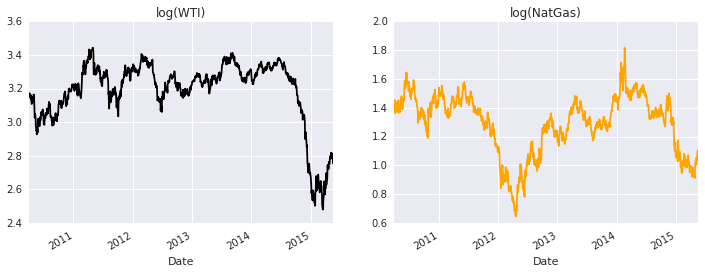#### Analysis¶ The spread between the two series has definitely increased when compared to the past. I chose 04-2010 as the starting point to test Granger Causality because that is when the relationship between the two series seem to decouple. In : %R print(VARselect(y.post,lag=20,type='const')$selection) #step 4) Determining maximum lag length

AIC(n)  HQ(n)  SC(n) FPE(n)
2      1      1      2

In :
#Either lag 1 or 2
#step 3) specifying a VAR model on the levels
#Step 5) Making sure the VAR model is well specified:
%R v_1 <- VAR(y.post,p=1,type='const')
%R v_2 <- VAR(y.post,p=2,type='const') #lag 2

%R print(serial.test(v_1,lags.bg=16,type='BG'))
%R print(serial.test(v_2,lags.bg=16,type='BG'))

	Breusch-Godfrey LM test

data:  Residuals of VAR object v_1
Chi-squared = 67.524, df = 64, p-value = 0.3577


	Breusch-Godfrey LM test

data:  Residuals of VAR object v_2
Chi-squared = 59.97, df = 64, p-value = 0.6197



#### Analysis¶

We can see that both the lag 1 and lag 2 show no serial correlation at the residuals. This is confirmed by the Breusch-Godfrey Lagrange Multiplier Test with a p value of 0.3577 and 0.6197. I will choose a lag 2 model based on AIC criteria. Hence we choose lag-2:

In :
%R print(coef(v_2))

$log.natgas. Estimate Std. Error t value Pr(>|t|) log.natgas..l1 0.926942866 0.02800107 33.1038318 2.147082e-174 log.wti..l1 0.011203670 0.04159033 0.2693816 7.876792e-01 log.natgas..l2 0.064148432 0.02799846 2.2911415 2.211609e-02 log.wti..l2 -0.007206275 0.04166948 -0.1729389 8.627266e-01 const -0.001546080 0.01271948 -0.1215521 9.032727e-01$log.wti.
Estimate  Std. Error    t value      Pr(>|t|)
log.natgas..l1 -0.013841171 0.018871576 -0.7334401  4.634235e-01
log.wti..l1     0.936408609 0.028030176 33.4071615 9.390538e-177
log.natgas..l2  0.013269881 0.018869813  0.7032333  4.820375e-01
log.wti..l2     0.061332785 0.028083523  2.1839420  2.914639e-02
const           0.007613573 0.008572405  0.8881489  3.746265e-01


In :
#stability analysis
print(robj.r('1/roots(v_2)[]')) # > 1
print(robj.r('1/roots(v_2)[]')) # > 1
#alternative stability analysis,
#Computes an empirical fluctuation process

%R stability_v2 <- stability(v_2)
%R plot(stability_v2)

 1.008164

 1.002454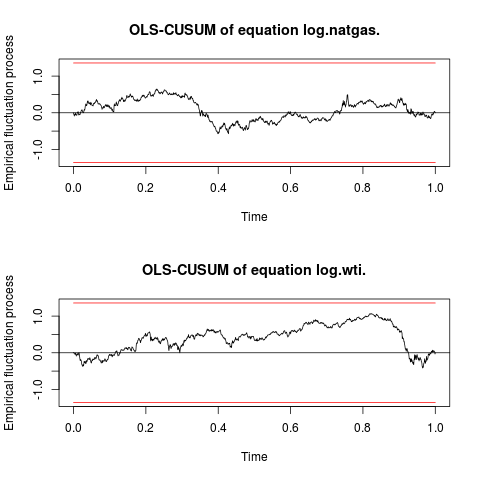In :
%R plot(stability(v_2,type='ME'))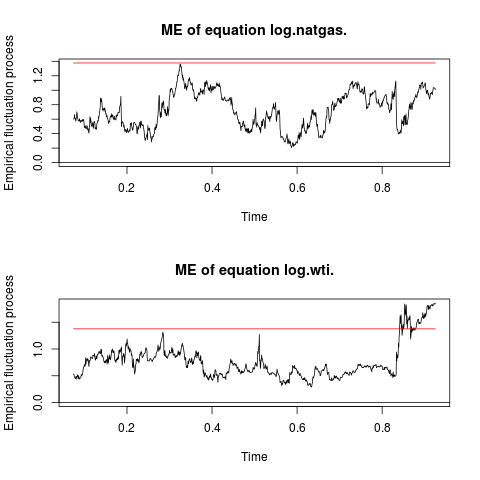In :
strucchange = importr('strucchange')
%R wt <- breakpoints(y.post[,2] ~ 1)
%R print(wt)
%R print(summary(wt))

	 Optimal 5-segment partition:

Call:
breakpoints.formula(formula = y.post[, 2] ~ 1)

Breakpoints at observation number:
225 535 807 1101

Corresponding to breakdates:
225 535 807 1101

	 Optimal (m+1)-segment partition:

Call:
breakpoints.formula(formula = y.post[, 2] ~ 1)

Breakpoints at observation number:

m = 1                   1101
m = 2   194             1101
m = 3   194         819 1101
m = 4   225     535 807 1101
m = 5   194 405 623 817 1101

Corresponding to breakdates:

m = 1                   1101
m = 2   194             1101
m = 3   194         819 1101
m = 4   225     535 807 1101
m = 5   194 405 623 817 1101

Fit:

m   0        1        2        3        4        5
RSS    48.56    24.88    19.18    18.22    17.67    18.00
BIC  -562.76 -1414.17 -1736.90 -1789.07 -1814.81 -1775.89

In :
robj.r('''ocus2 <- efp(y.post[,2]~1,type='OLS-CUSUM')''')
robj.r('''ocus1 <- efp(y.post[,1]~1,type='OLS-CUSUM')''')
%R plot(ocus2)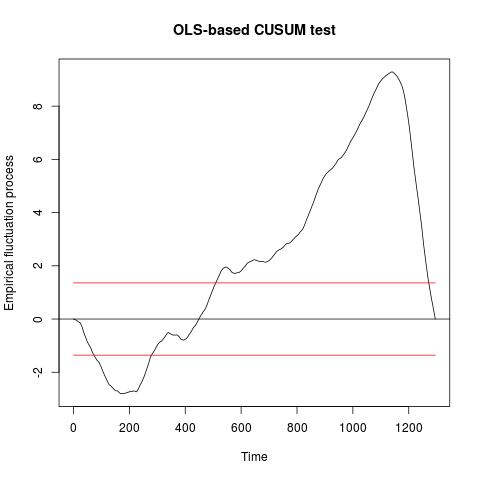In :
%R plot(ocus1)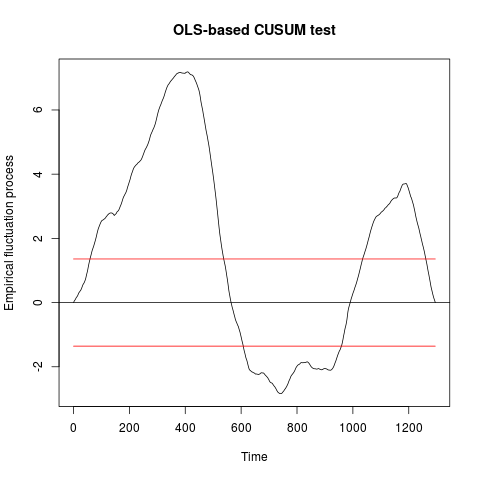In [ ]:


In :
rcParams['figure.figsize'] = 9,4
%R fm0 <- lm(y.post[,2] ~ 1)
%R fm1 <- lm(y.post[,2]~breakfactor(wt,breaks=4))
fitted_fm0 = %R fitted(fm0)
fitted_fm1 = %R fitted(fm1)
df_post['log(wti)'].plot(color='black')
plt.plot(df_post.index,fitted_fm1,color='orange')
fir = pd.to_datetime('2011-02-22')
sec = pd.to_datetime('2012-05-15')
third = pd.to_datetime('2013-06-13')
fourth = pd.to_datetime('2014-08-13')
plt.plot((fir,fir),(2.4,3.5),'b--')
plt.plot((sec,sec),(2.4,3.5),'b--')
plt.plot((third,third),(2.4,3.5),'b--')
plt.plot((fourth,fourth),(2.4,3.5),'b--')
plt.title('wti post')

Out:
<matplotlib.text.Text at 0x7fde0f938d50>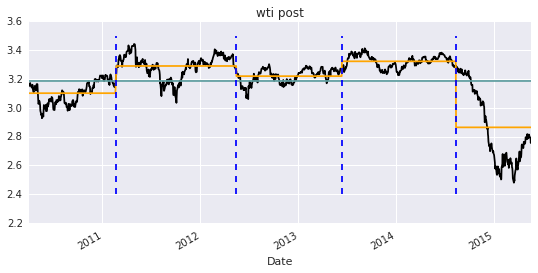In [ ]:


In :
%R wt <- breakpoints(y.pre[,2] ~ 1)
%R print(wt)
%R print(summary(wt))

	 Optimal 5-segment partition:

Call:
breakpoints.formula(formula = y.pre[, 2] ~ 1)

Breakpoints at observation number:
456 1220 1676 2361

Corresponding to breakdates:
456 1220 1676 2361

	 Optimal (m+1)-segment partition:

Call:
breakpoints.formula(formula = y.pre[, 2] ~ 1)

Breakpoints at observation number:

m = 1            1628
m = 2   456      1644
m = 3   456      1630      2360
m = 4   456 1220 1676      2361
m = 5   456 1078 1534 1990 2446

Corresponding to breakdates:

m = 1            1628
m = 2   456      1644
m = 3   456      1630      2360
m = 4   456 1220 1676      2361
m = 5   456 1078 1534 1990 2446

Fit:

m   0      1      2      3      4      5
RSS 1058.8  287.0  164.2  138.3  128.7  130.4
BIC 5441.4 1481.0 -202.6 -709.4 -913.7 -857.1

In :
df_pre.index[[456,1220,1676,2361]]

Out:
DatetimeIndex(['1999-10-27', '2002-11-20', '2004-09-21', '2007-06-15'], dtype='datetime64[ns]', name=u'Date', freq=None, tz=None)
In :
%R fm0 <- lm(y.pre[,2] ~ 1)
%R fm1 <- lm(y.pre[,2]~breakfactor(wt,breaks=4))
fitted_fm0 = %R fitted(fm0)
fitted_fm1 = %R fitted(fm1)
df_pre['log(wti)'].plot(color='black')
plt.plot(df_pre.index,fitted_fm0,color='green')
plt.plot(df_pre.index,fitted_fm1,color='red')
fir = pd.to_datetime('1999-10-27')
sec = pd.to_datetime('2002-11-20')
third = pd.to_datetime('2004-09-21')
fourth = pd.to_datetime('2007-06-15')
plt.plot((fir,fir),(1.0,3.5),'b--')
plt.plot((sec,sec),(1.0,3.5),'b--')
plt.plot((third,third),(1.0,3.5),'b--')
plt.plot((fourth,fourth),(1.0,3.5),'b--')
plt.plot(df_pre.index,df_pre['log(natgas)'].values,color='orange')

Out:
[<matplotlib.lines.Line2D at 0x7fde0f4f8e90>]